## Quiz: Calculating Measurements of Basic Figures

Use the triangle below for questions 1 and 2.Use the rectangle below for questions 3 and 4.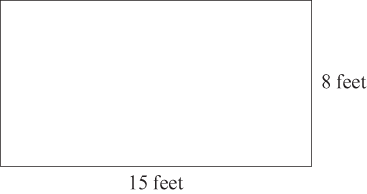Use the square below for questions 5 and 6.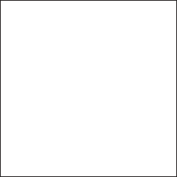Use the parallelogram below for questions 7 and 8.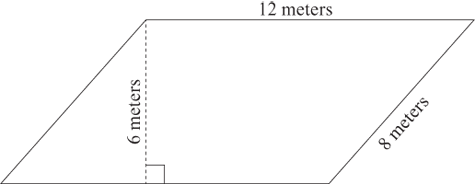Use the trapezoid below for questions 9 and 10.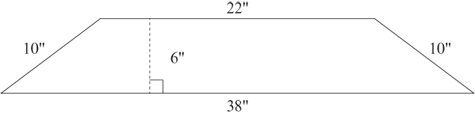Use the circle below for questions 11 and 12.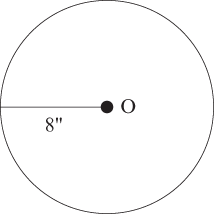Use the figures below to answer questions 13 and 14.Use the figures below to answer questions 15 and 16.